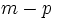# Normal bundle of a submanifold

## Definition

### Definition for differential manifolds

Let$M$ be a differential manifold (of dimension$m$) and$P$ a submanifold (of dimension$p$) of$M$. Then:

1. The tangent bundle$TM$ restricts to a vector bundle over$P$. This is a bundle with fibers having dimension$n$
2. The tangent bundle$TP$ is a$p$-dimensional vector bundle over$P$. This is a subbundle of the preceding bundle.

The quotient of the first bundle by the second is termed the normal bundle to$P$ in$M$. It is a vector bundle with fibers having dimension$m - p$.

### Definition for Riemannian manifolds

Let$M$ be a Riemannian manifold and$P$ be a submanifold. We have two bundles:

1. The tangent bundle$TM$ restricts to a vector bundle over$P$. This is a bundle with fibers having dimension$n$
2. The tangent bundle$TP$ is a$p$-dimensional vector bundle over$P$. This is a subbundle of the preceding bundle.

The normal bundle to$P$ in$M$ is defined as the orthogonal complement to bundle (2) in bundle (1).

There is a natural isomorphism between this and the normal bundle defined abstractly for Riemannian manifolds, based on the general fact that a direct sum complement to a subbundle, is isomorphic to the quotient.